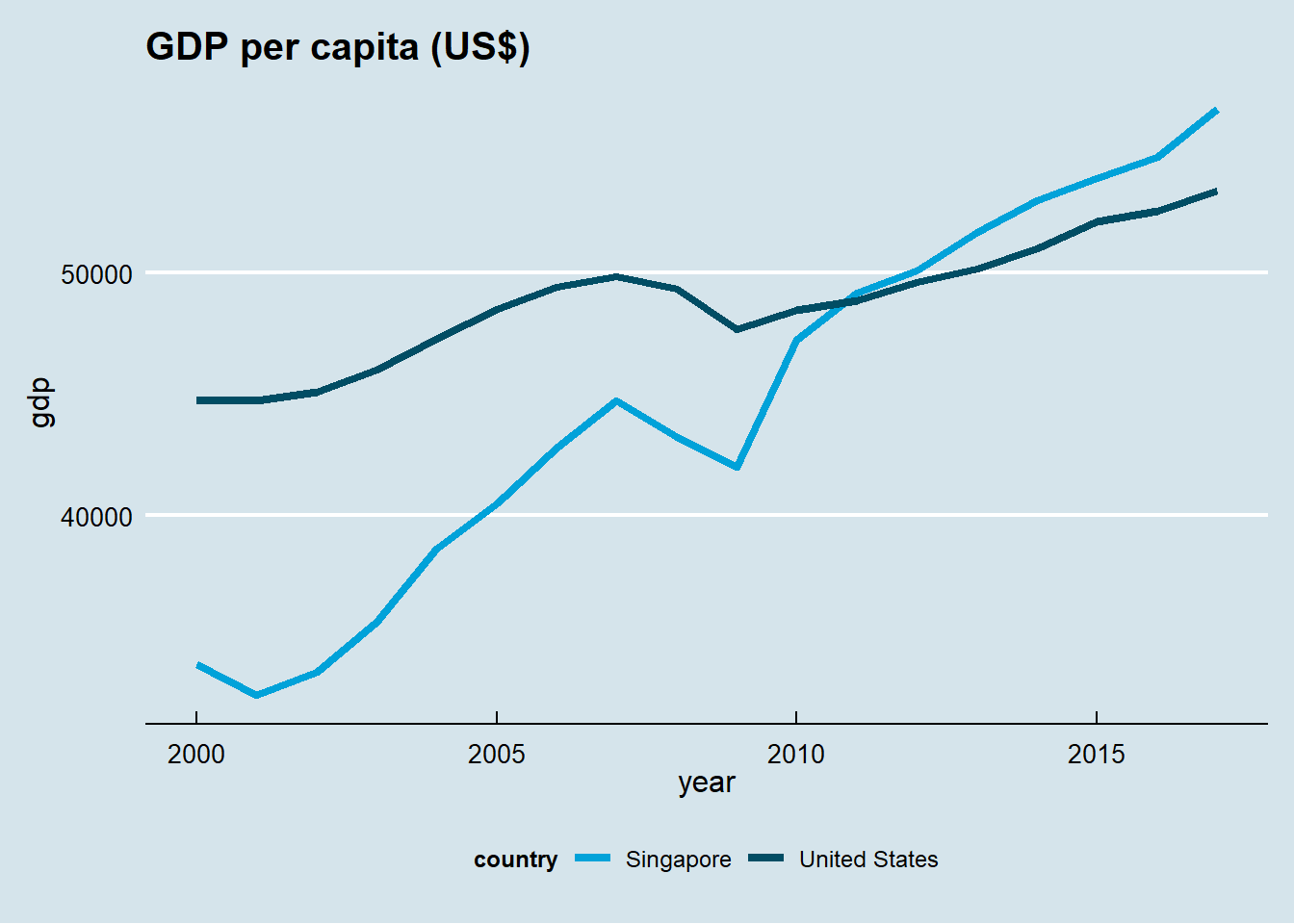## 11.1 ggplot2 package

ggplot2 is a powerful package to draw graphics. It implements the grammar of graphics (and hence its name).

install.packages("ggplot2")
library(ggplot2)

Two plotting functions in the package:

1. qplot() and
2. ggplot().

While qplot() is useful to plot quickly, most of time, one should use ggplot() for systemic plotting

qplot(x, y, dataframe, geom="type")
ggplot(data, aes(x,y))+ geom_type() + options

We illustrate based on the following three data series:

First, we have a vector of intgers from one to 30.

x<-1:30

Second, we have another vector of three random integers.

y<-c(5,4,5,3,4,6,7,4,2,1,5,6,2,2,2,
5,6,7,8,6,2,8,7,8,8,3,3,7,2,1)

Third, we have a vector of categorical variables:

z<-c('A','B','A','A','B','A','B','B','A','A',
'B','A','A','A','B','A','A','B','A','B',
'B','A','A','A','B','A','A','B','A','B')
df<-data.frame(x,y,z)

### 11.1.1 Qplot

Scatter plot is useful to depict relationship between two variables.

qplot(x, y, data=df, geom="point")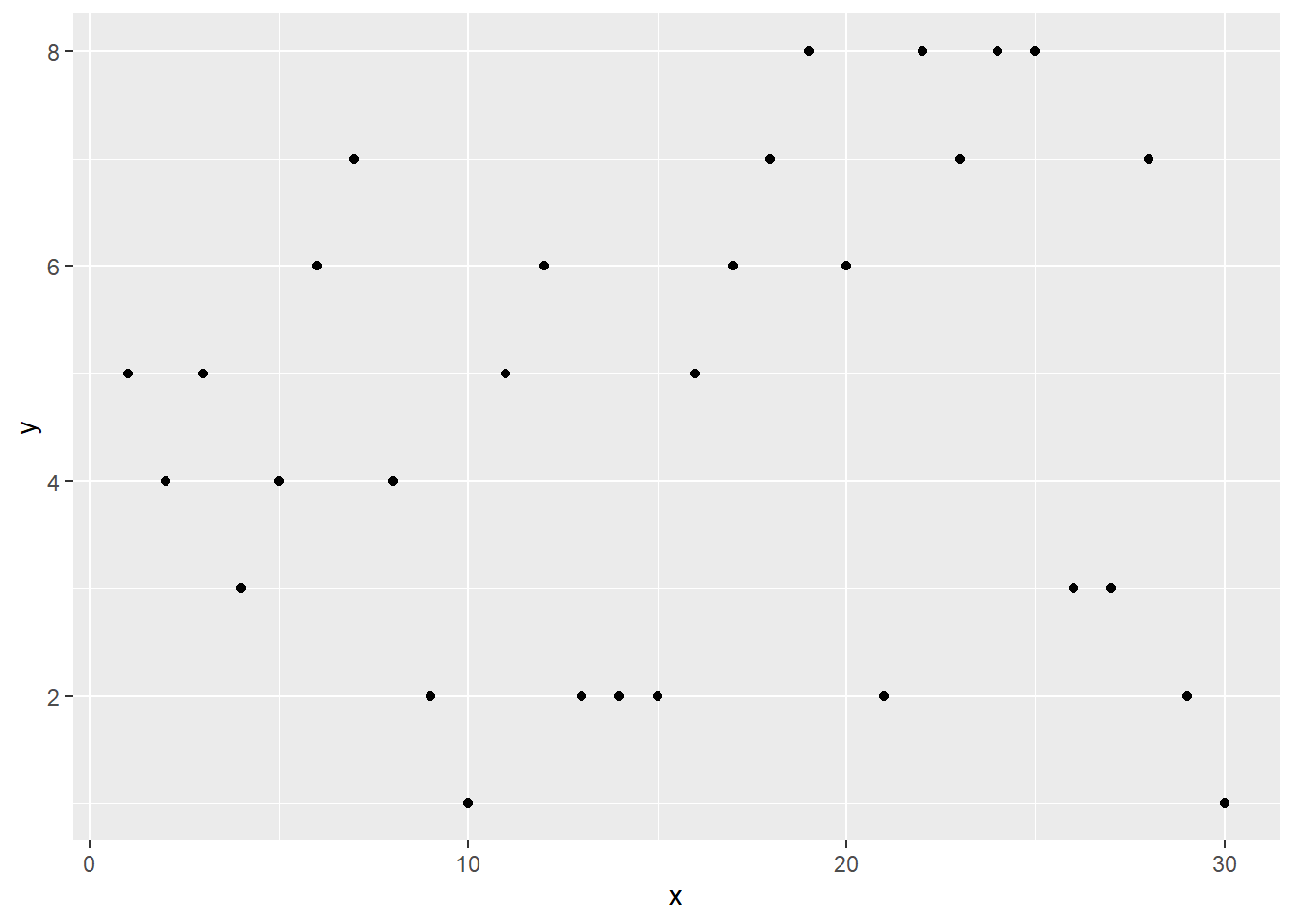Figure 11.1: Scatter plot

Box plot: use when one variable is continuous, another is categorical (discrete). Then box plot is an alternative to scatter plot.

qplot(z,x, data=df, geom="boxplot")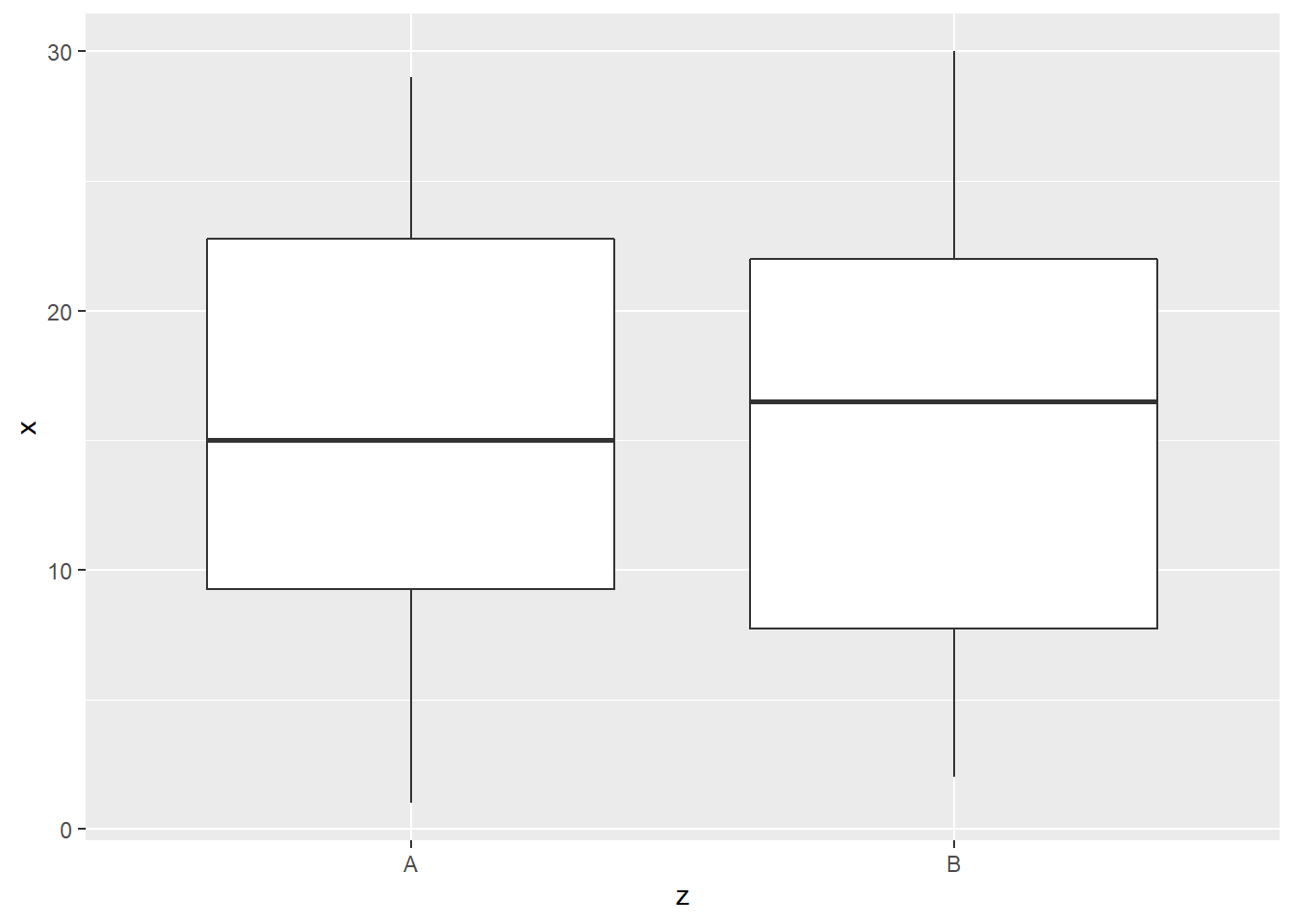Figure 11.2: Box plot

Line plot is useful to show time trend and how two variables are related. Compared to scatter plot, line plot is most useful if the horizontal variable does not have any duplicated values.

qplot(x, y, data=df, geom="line")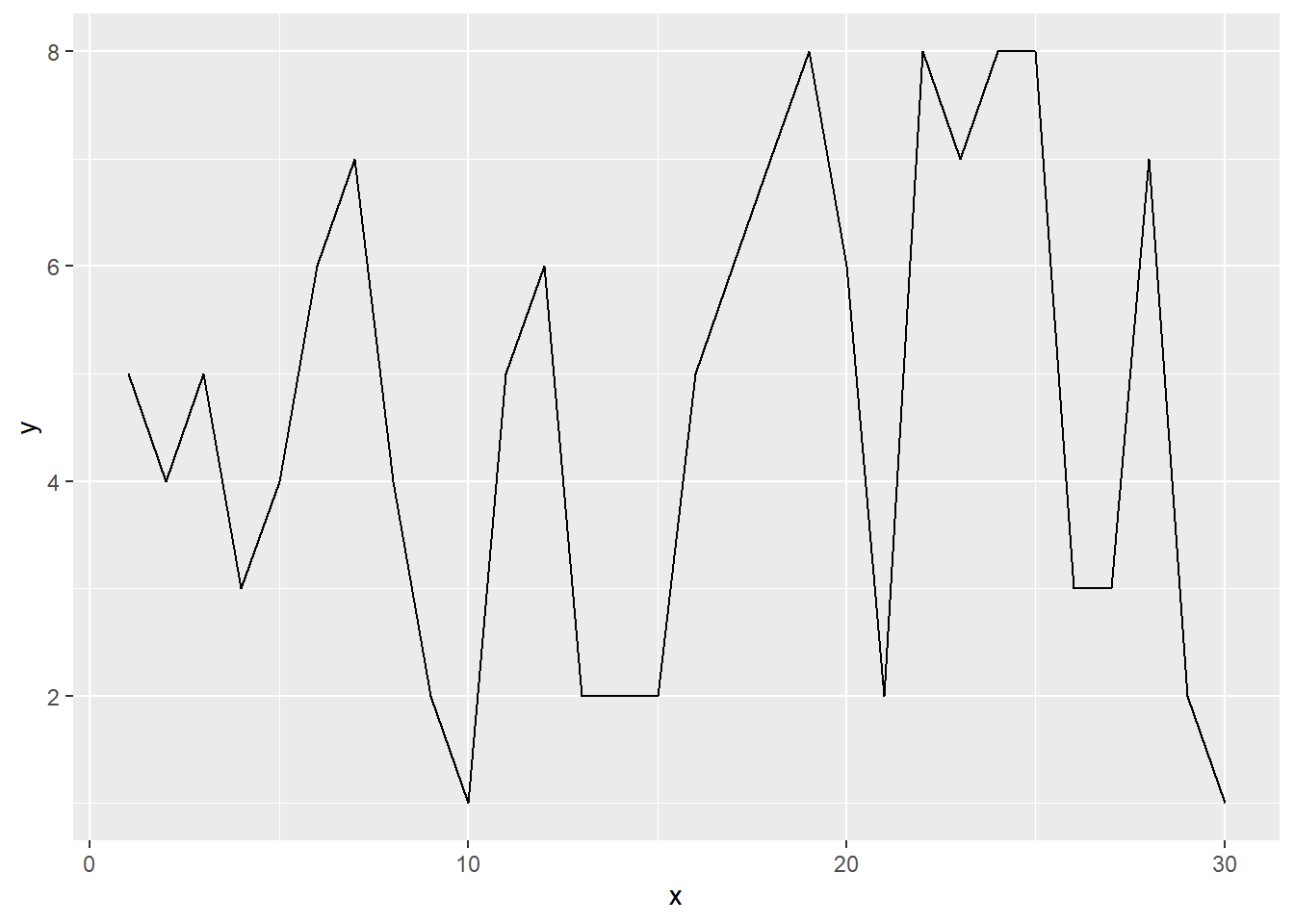Figure 11.3: Line plot

Histogram is useful if we want to visualize the distribution of single continuous variable.

qplot(y, data=df, geom="histogram",binwidth = 3)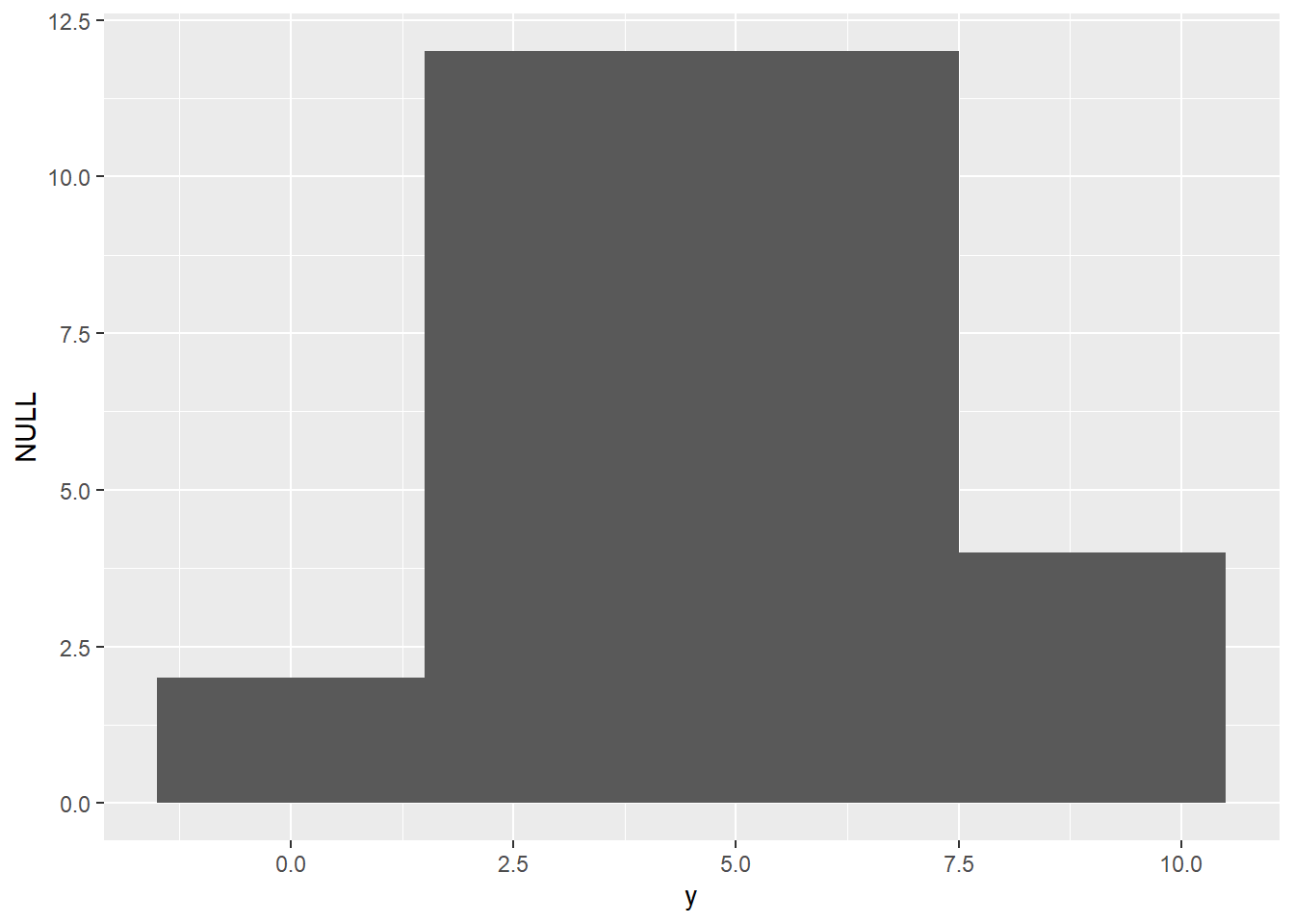Figure 11.4: Histogram

Density plot is similar to histogram but there is no grouping as in histogram but the function is smoothed.

qplot(y, data=df, geom="density")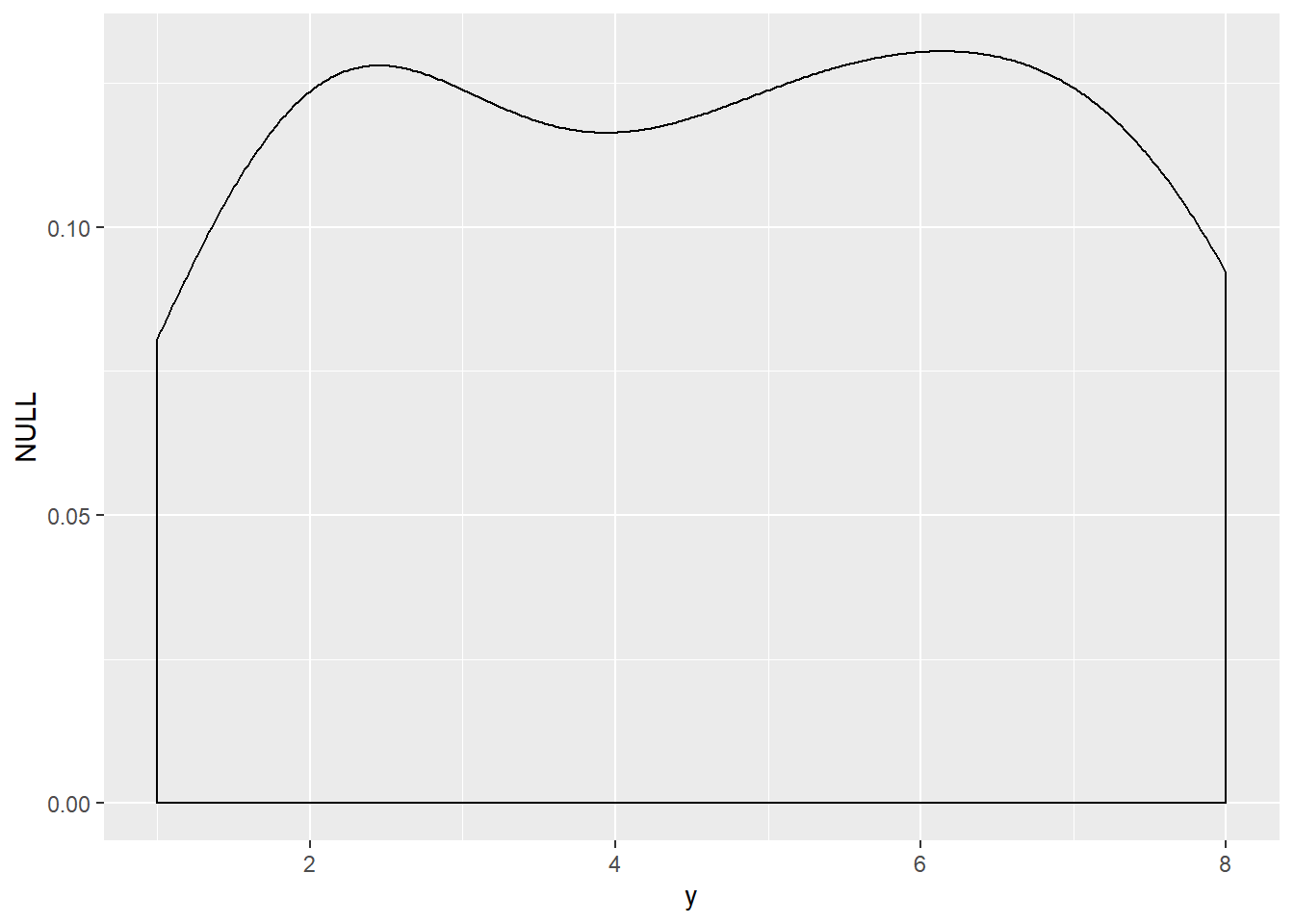Figure 11.5: Density plot

Bar chart is similar to histogram but it is for discrete data.

qplot(z, data=df, geom="bar")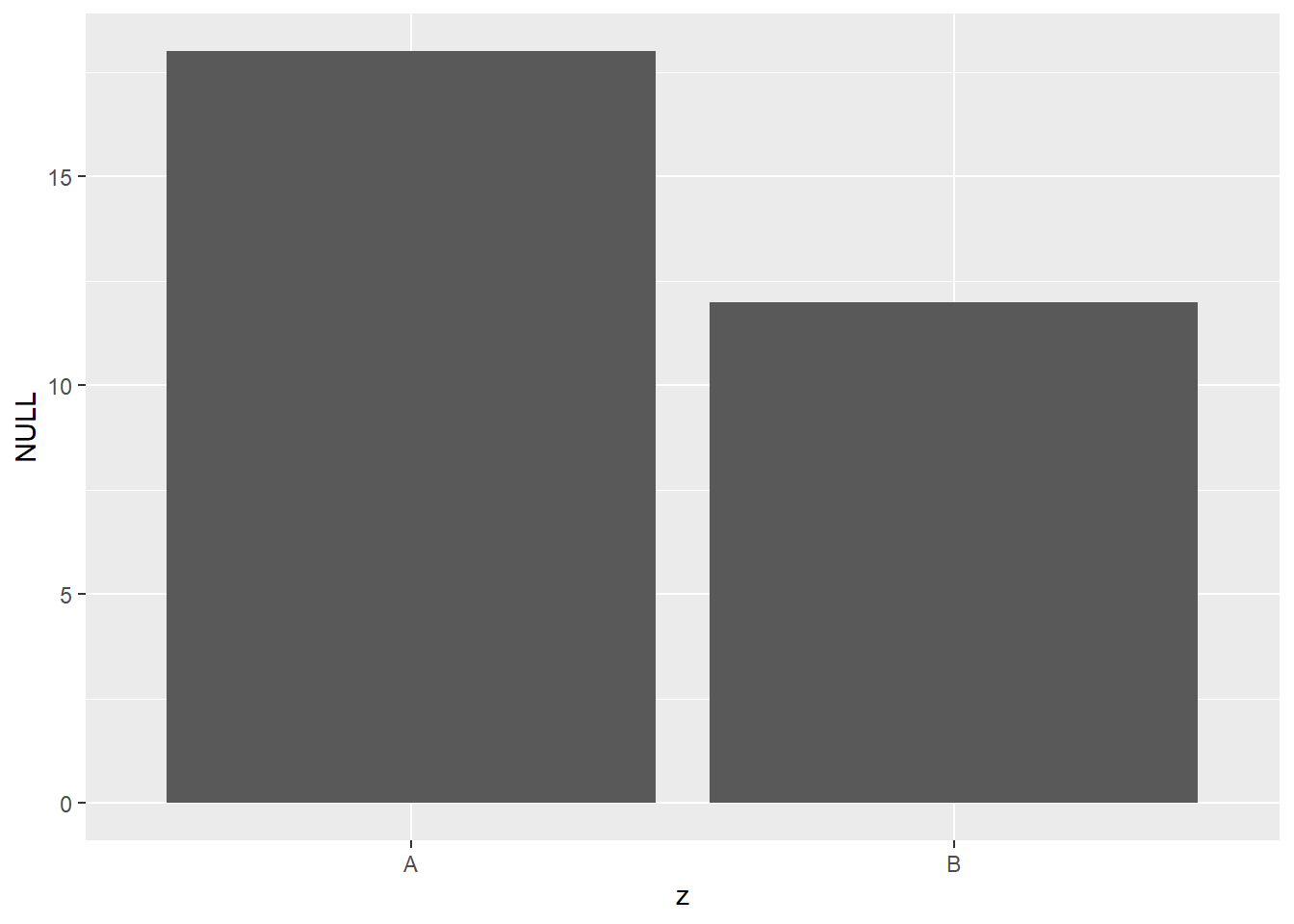Figure 11.6: Bar chart

### 11.1.2 ggplot

The syntax for base plots is as follows:

ggplot(data)+aes(x,y)+geom_point()
ggplot(data)+aes(x,y)+geom_boxplot()
ggplot(data)+aes(x,y)+geom_line()
ggplot(data)+aes(x)+geom_histogram()
ggplot(data)+aes(x)+geom_freqpoly()
ggplot(data)+aes(x)+geom_bar()

Scatter plot

ggplot(df)+aes(x,y)+geom_point()Figure 11.7: Scatter plot

Boxplot

ggplot(df)+aes(z,x)+geom_boxplot()Figure 11.8: Box plot

Lineplot

ggplot(df)+aes(x,y)+geom_line()Figure 11.9: Line plot

Histogram

ggplot(df)+aes(y)+geom_histogram(binwidth=1)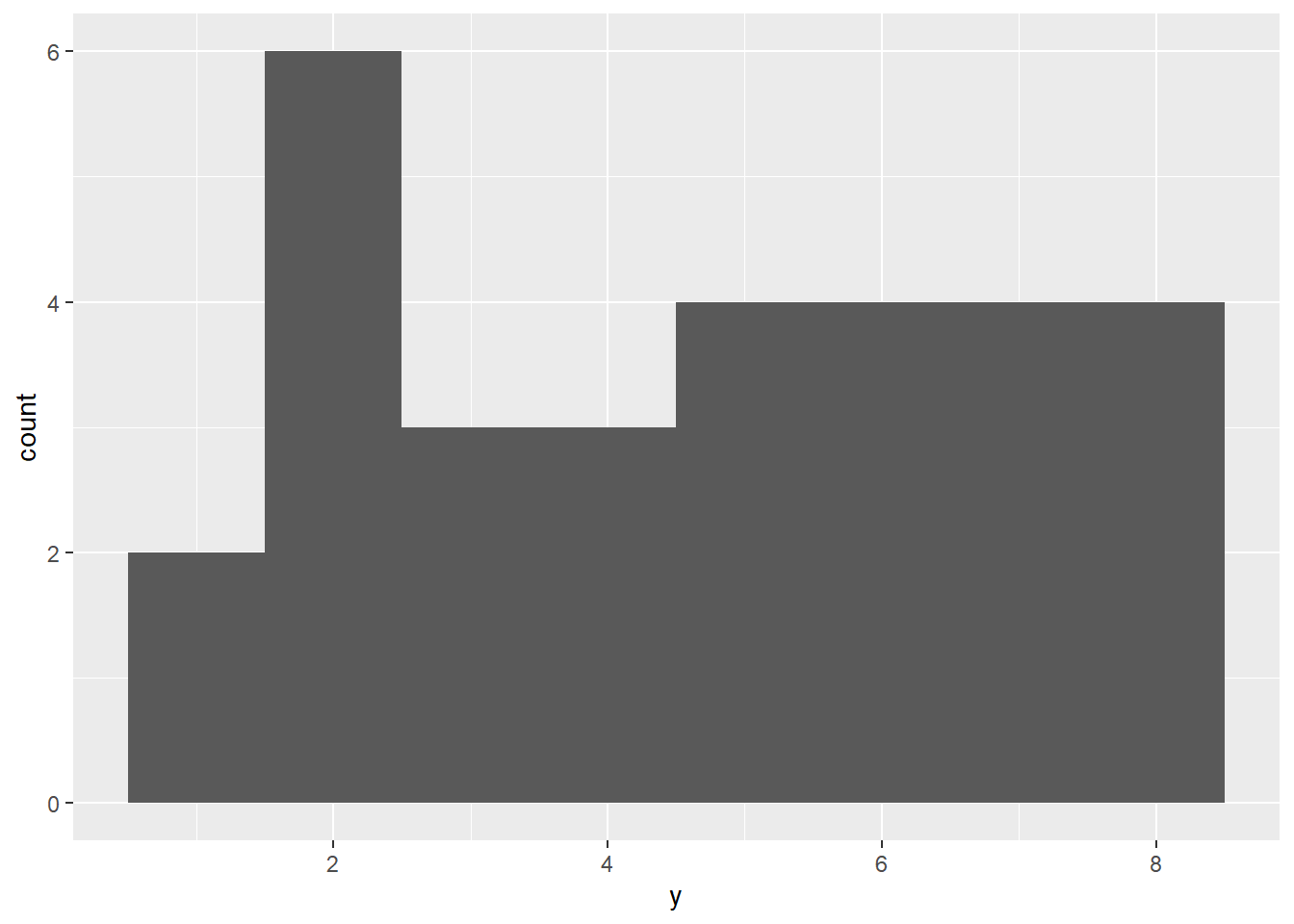Figure 11.10: Histogram

Frequency polygon

ggplot(df)+aes(y)+geom_freqpoly(binwidth=1)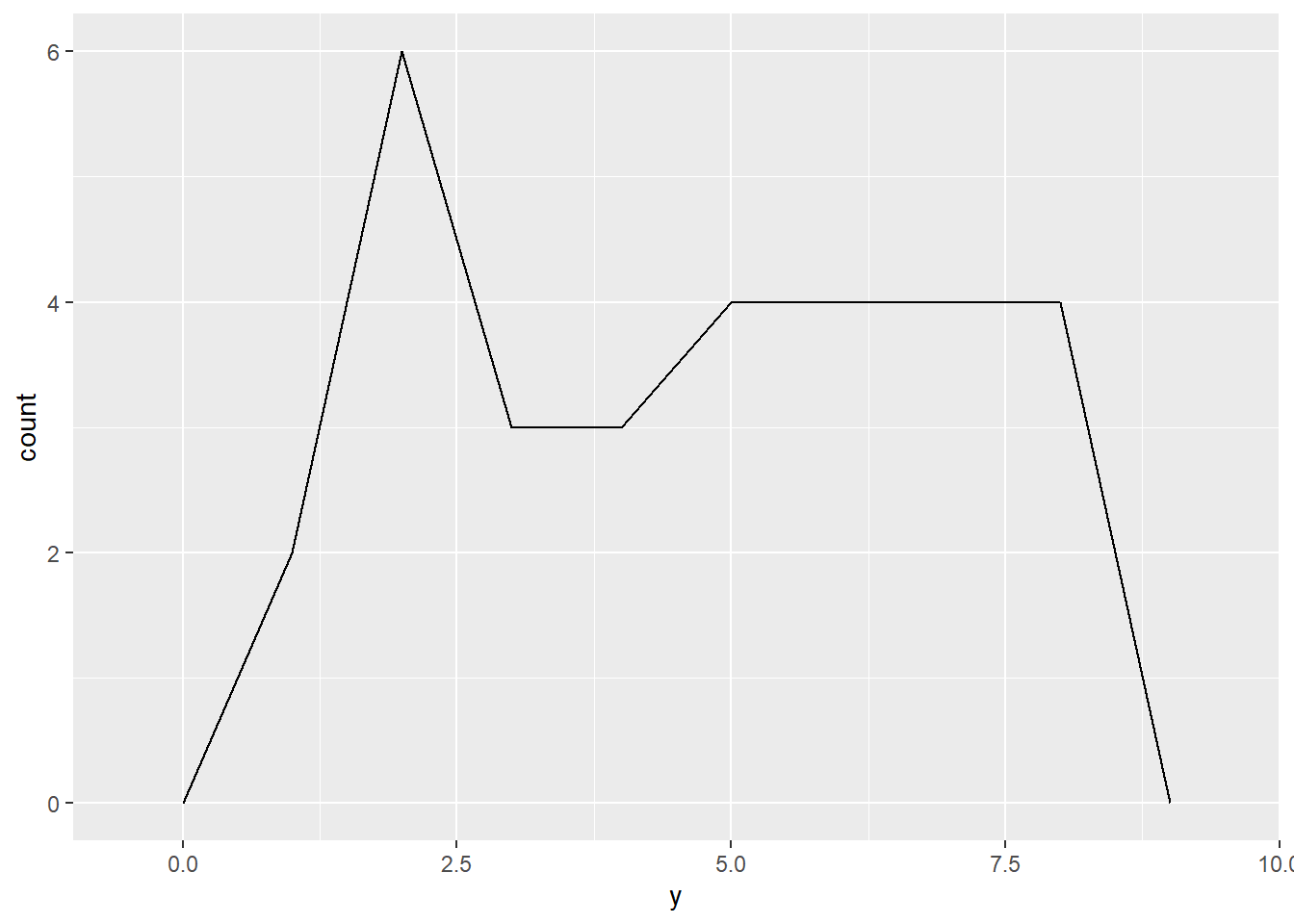Figure 11.11: Frequency polygon

Bar Chart

ggplot(df)+aes(y)+geom_bar()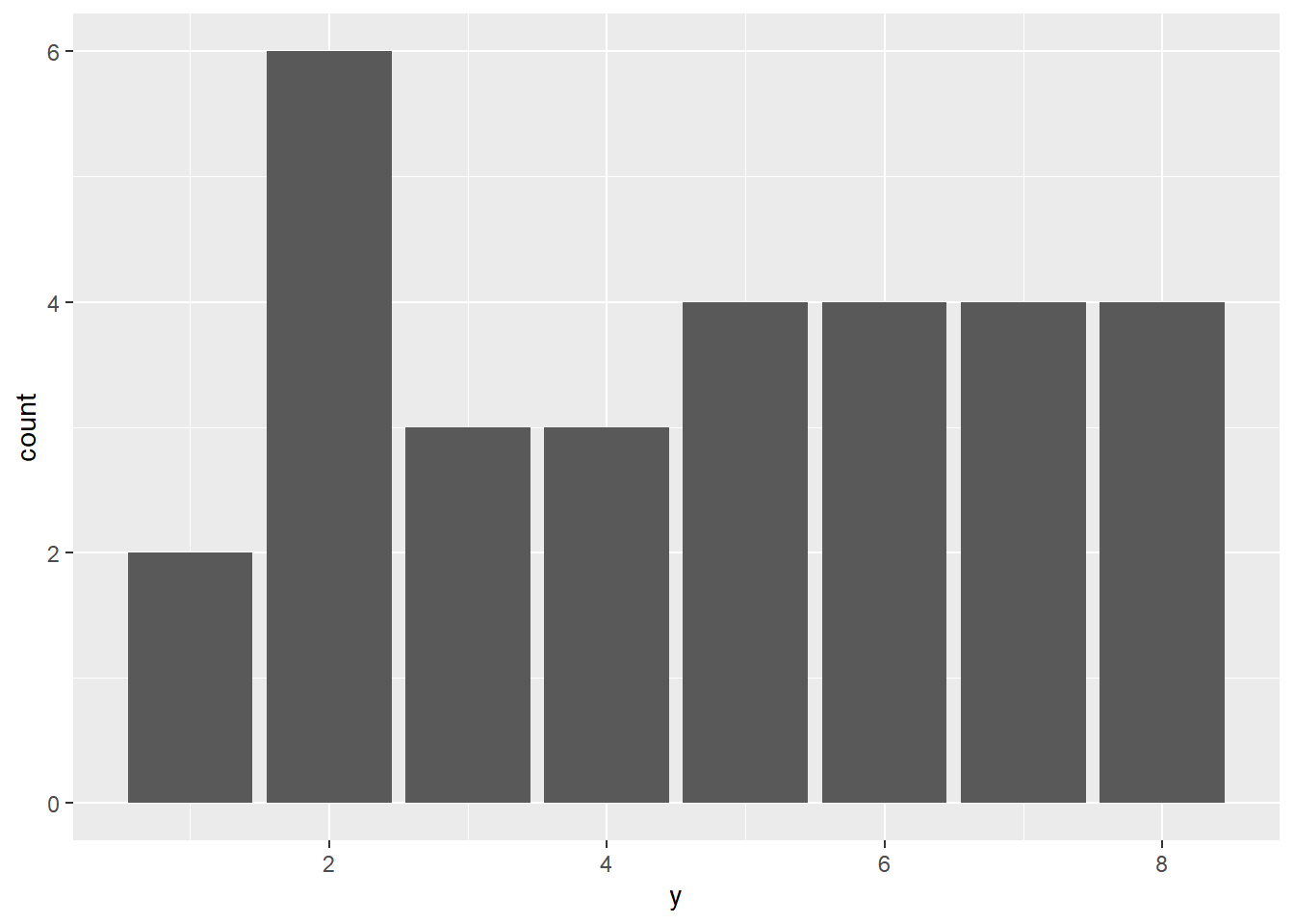Figure 11.12: Bar chart

### 11.1.3 Aesthetic

To improve the appearance, we can change color, shape (for scatter plot) and size (for scatter plot).

Color: aes(x,y, color=z)

ggplot(df) +aes(x,y, color=z) + geom_point()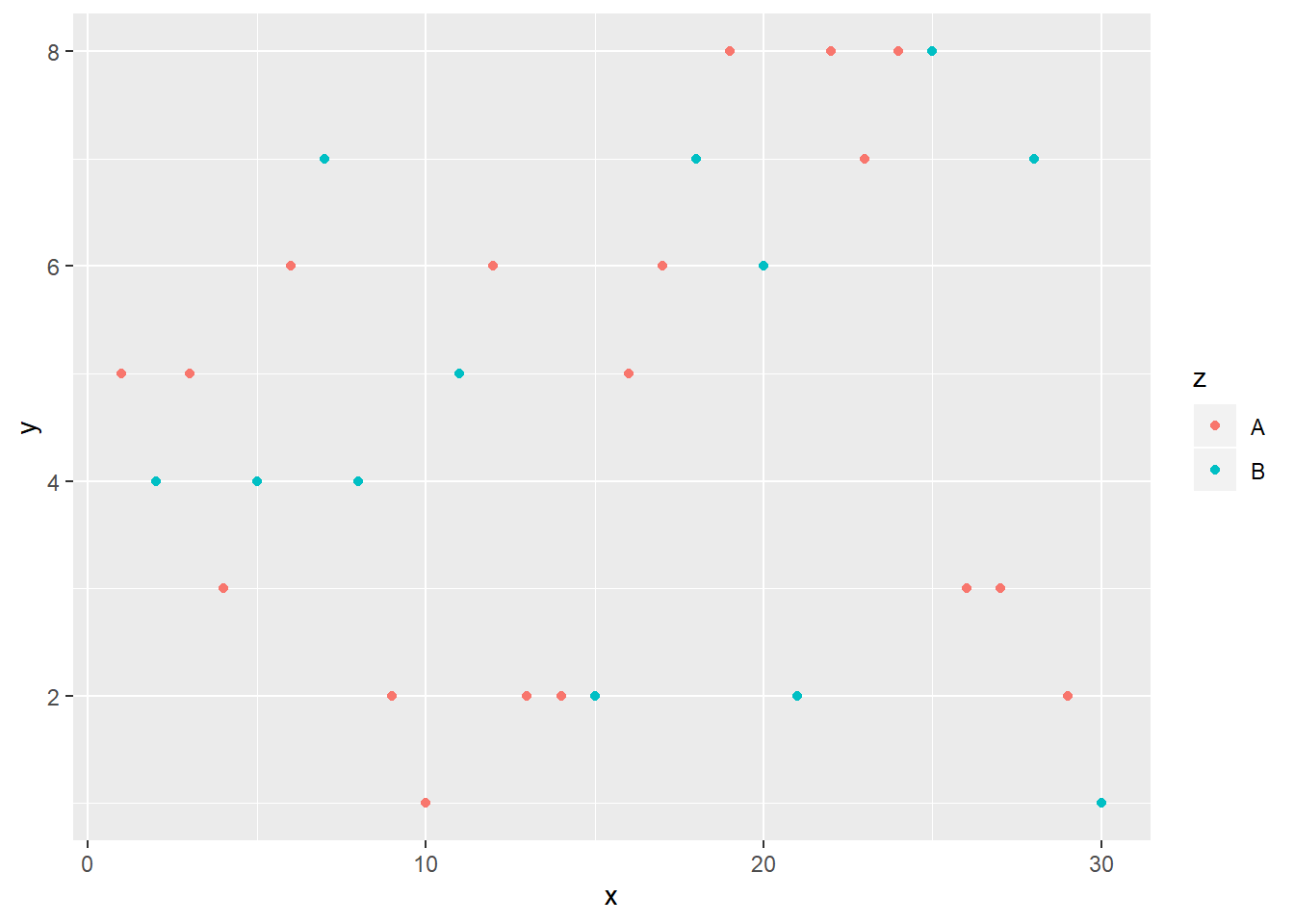shape: aes(x,y, shape=z)

ggplot(df) + aes(x,y, shape=z) + geom_point()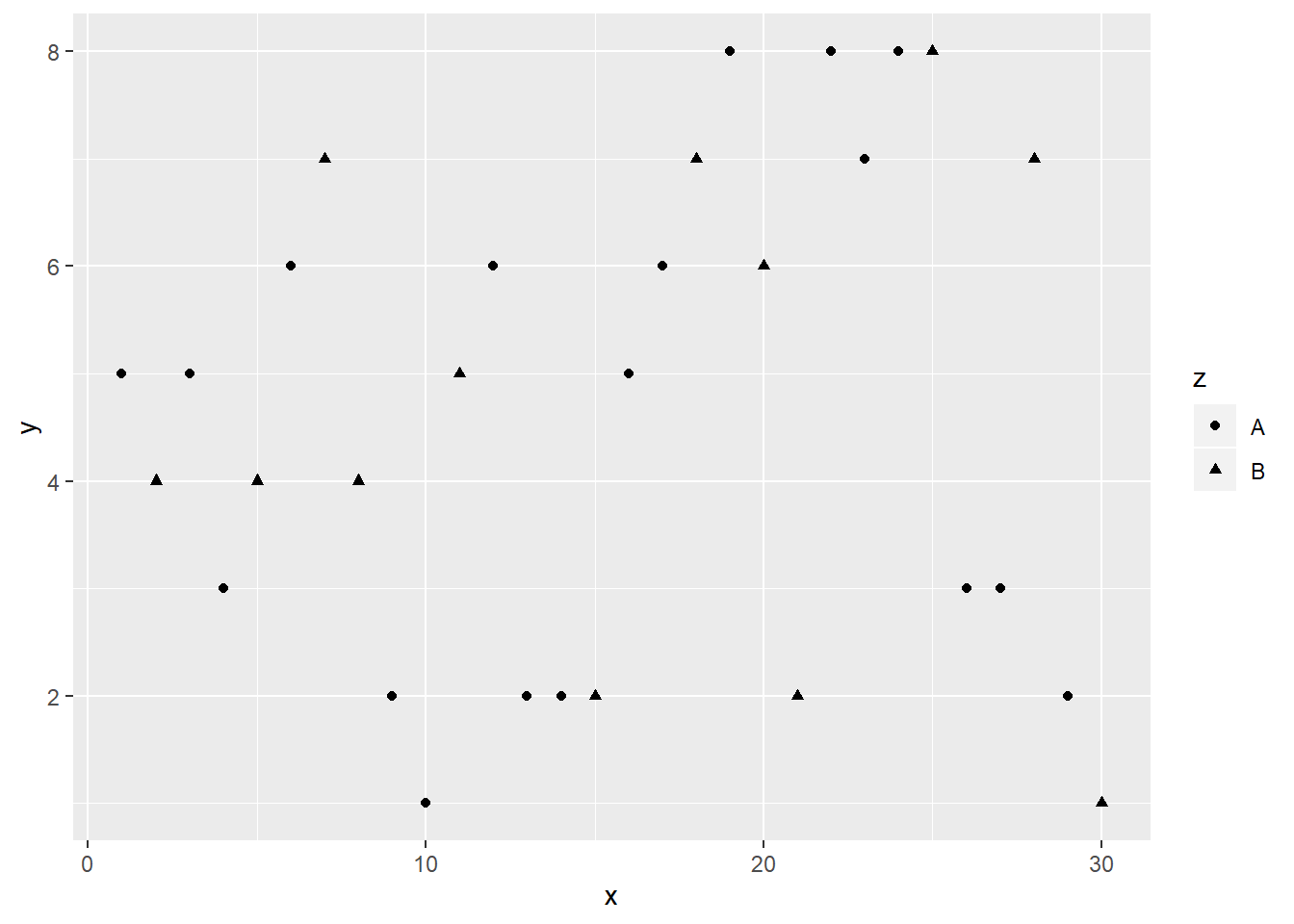Size: aes(x,y, size = 3)

ggplot(df) + aes(x,y, size = 3) + geom_point()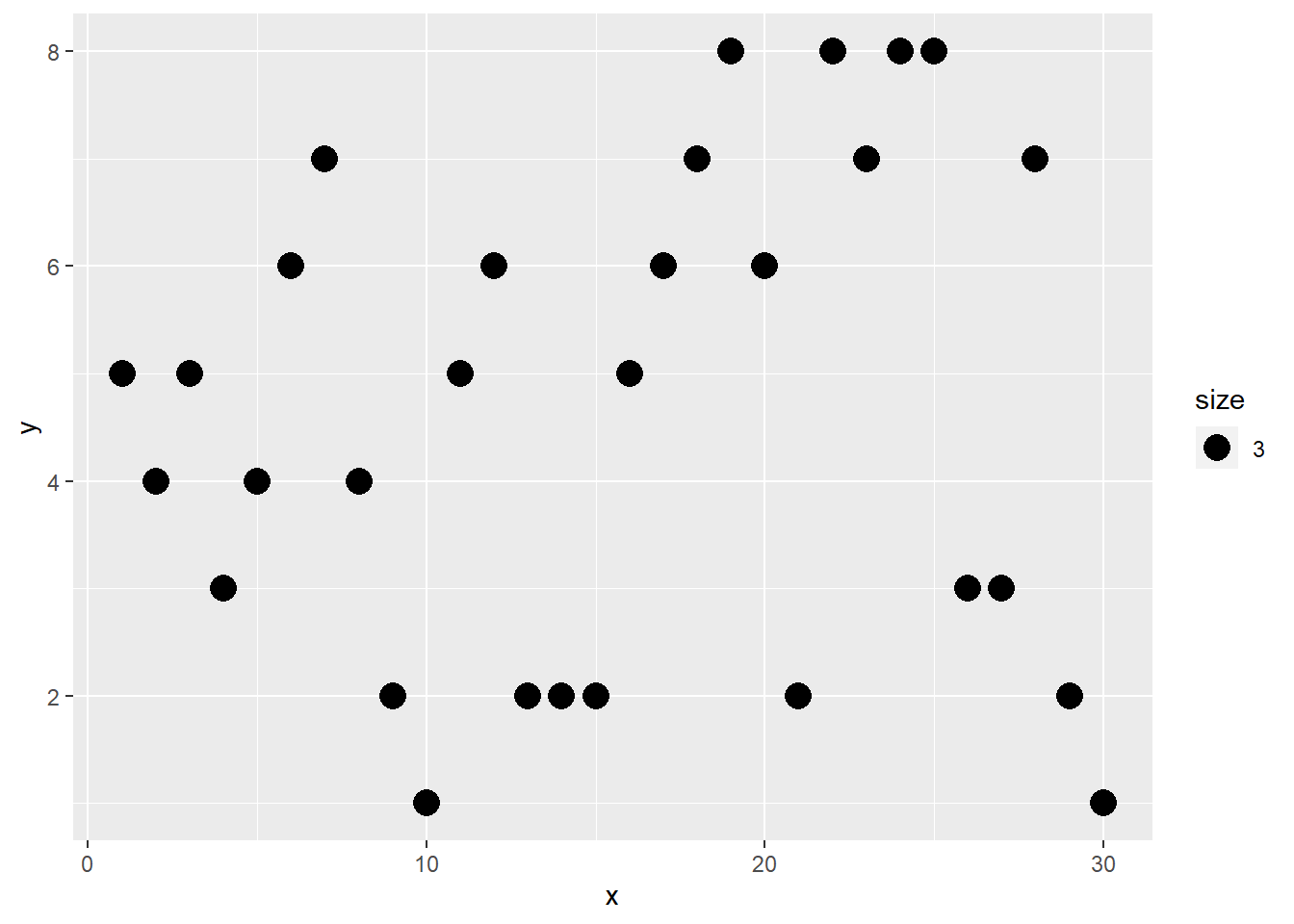### 11.1.4 Decoration

To improve the readability of plot, one may add title, label axes, and provide legend.

ggplot(df) + aes(x,y)+ geom_point()+
ggtitle("title")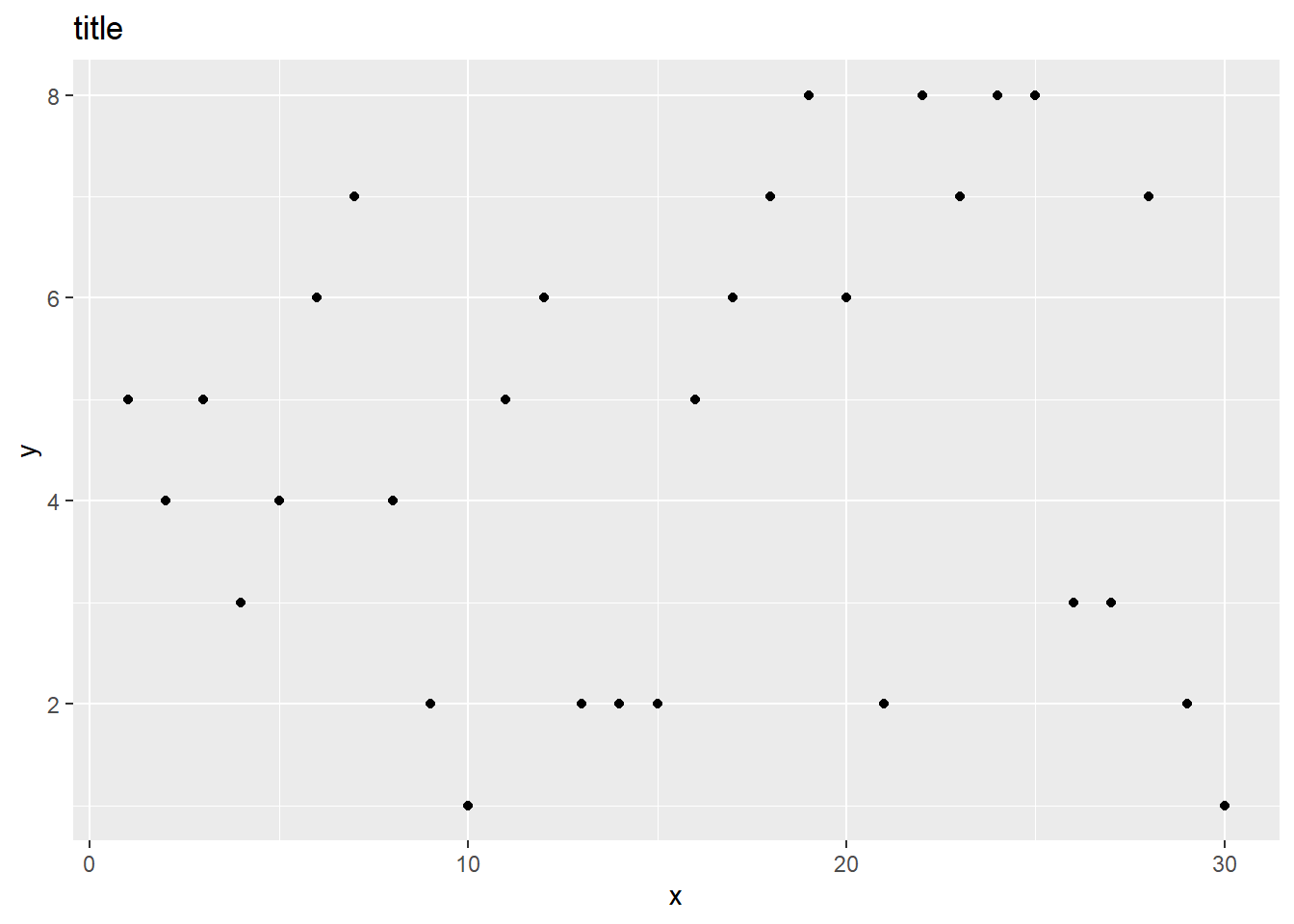Label Axes using xlab() and ylab().

ggplot(df) + aes(x,y)+ geom_point()+
xlab("X axis") + ylab("Y axis")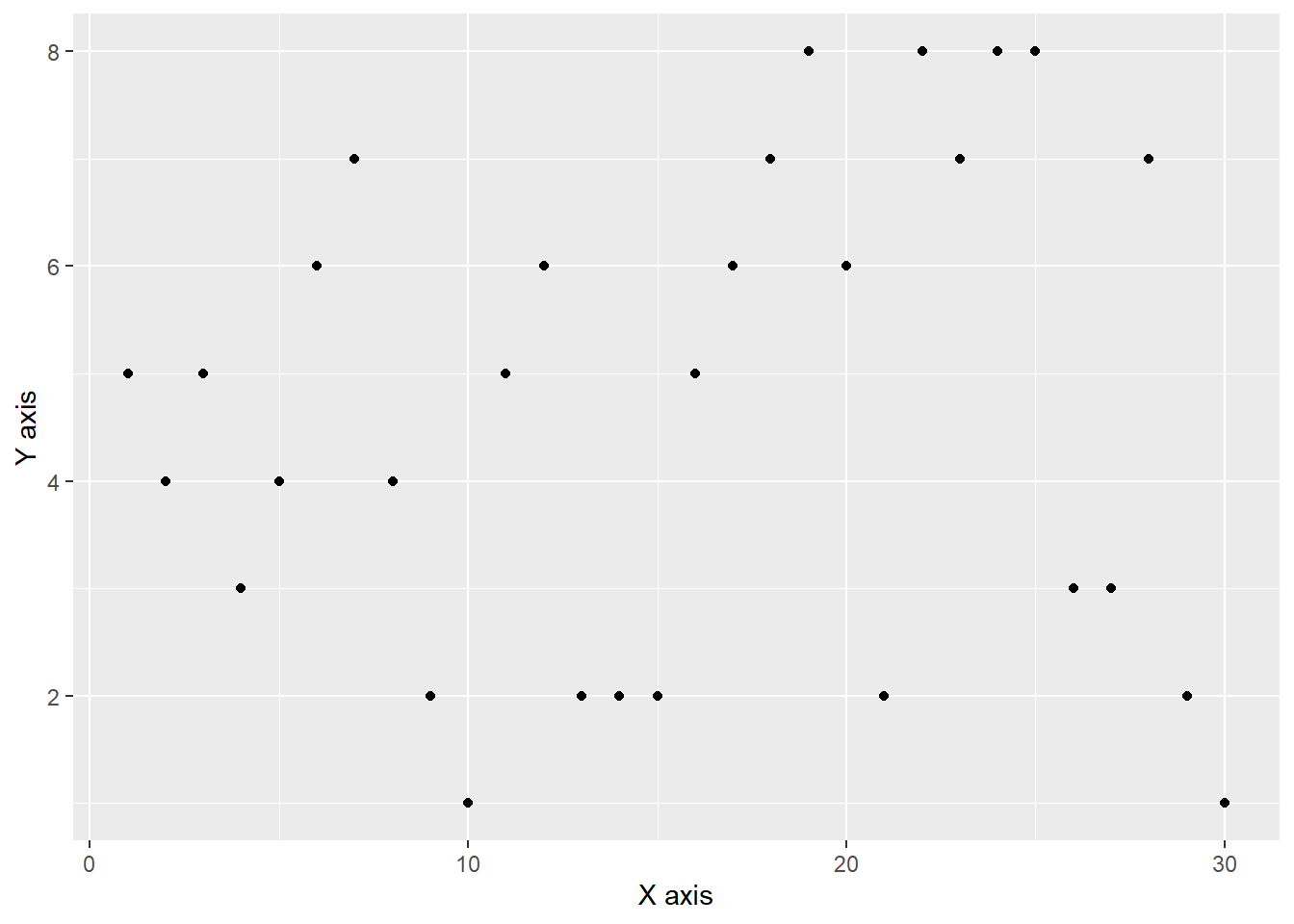Legend can be added using theme(legend). The position can be top, right, left and bottom.

ggplot(df) + aes(x,y,color=z)+geom_point()+
theme(legend.position = "bottom")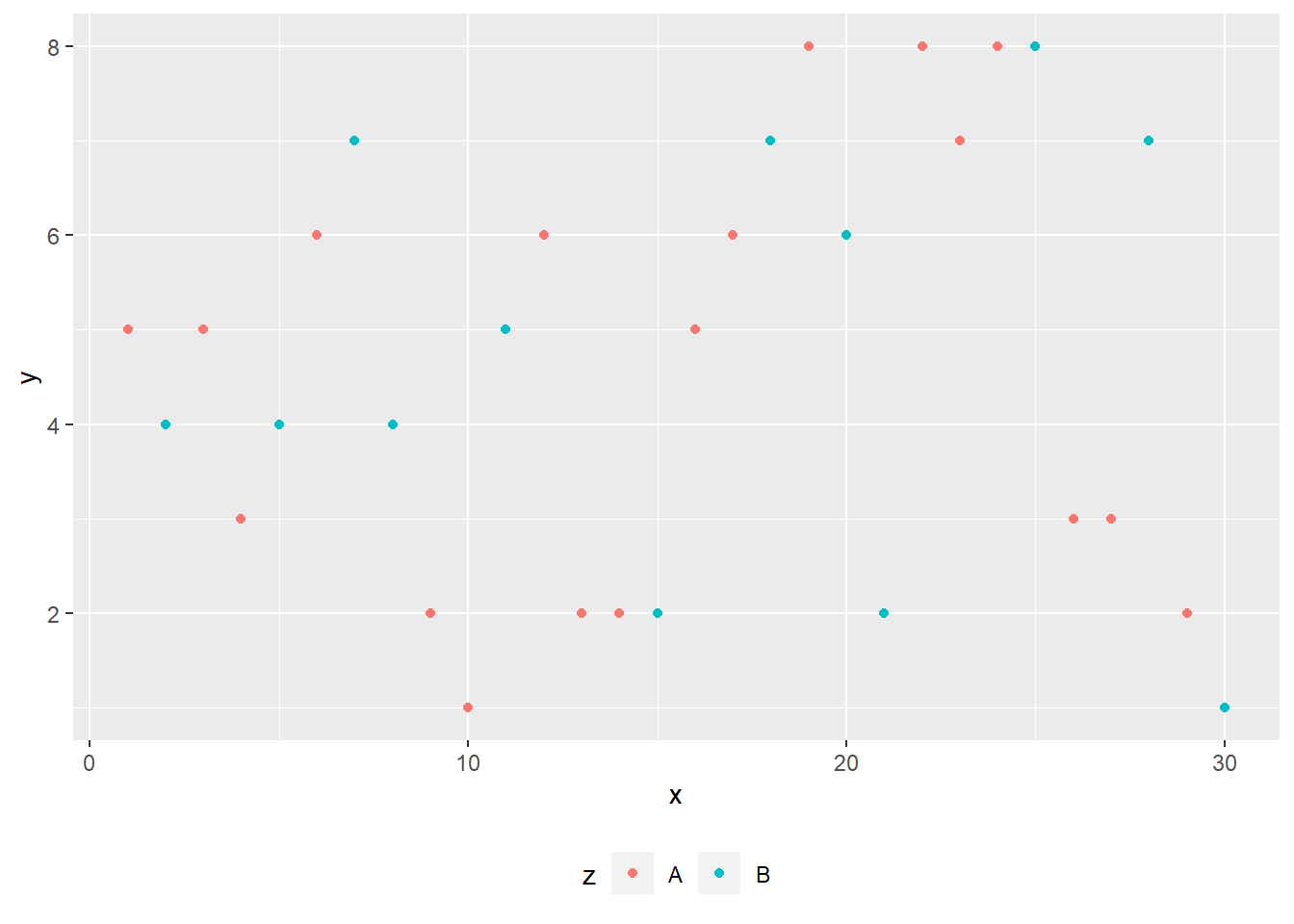### 11.1.5 Multiple Plots

It is often that we put plot side by side or even in a grid. We can use facet_wrap() to make multiple graphs.

The following code makes the two plots side by side:

ggplot(data=df)+
aes(x,y, color=z)+
geom_point()+
facet_wrap(~ z, ncol = 2)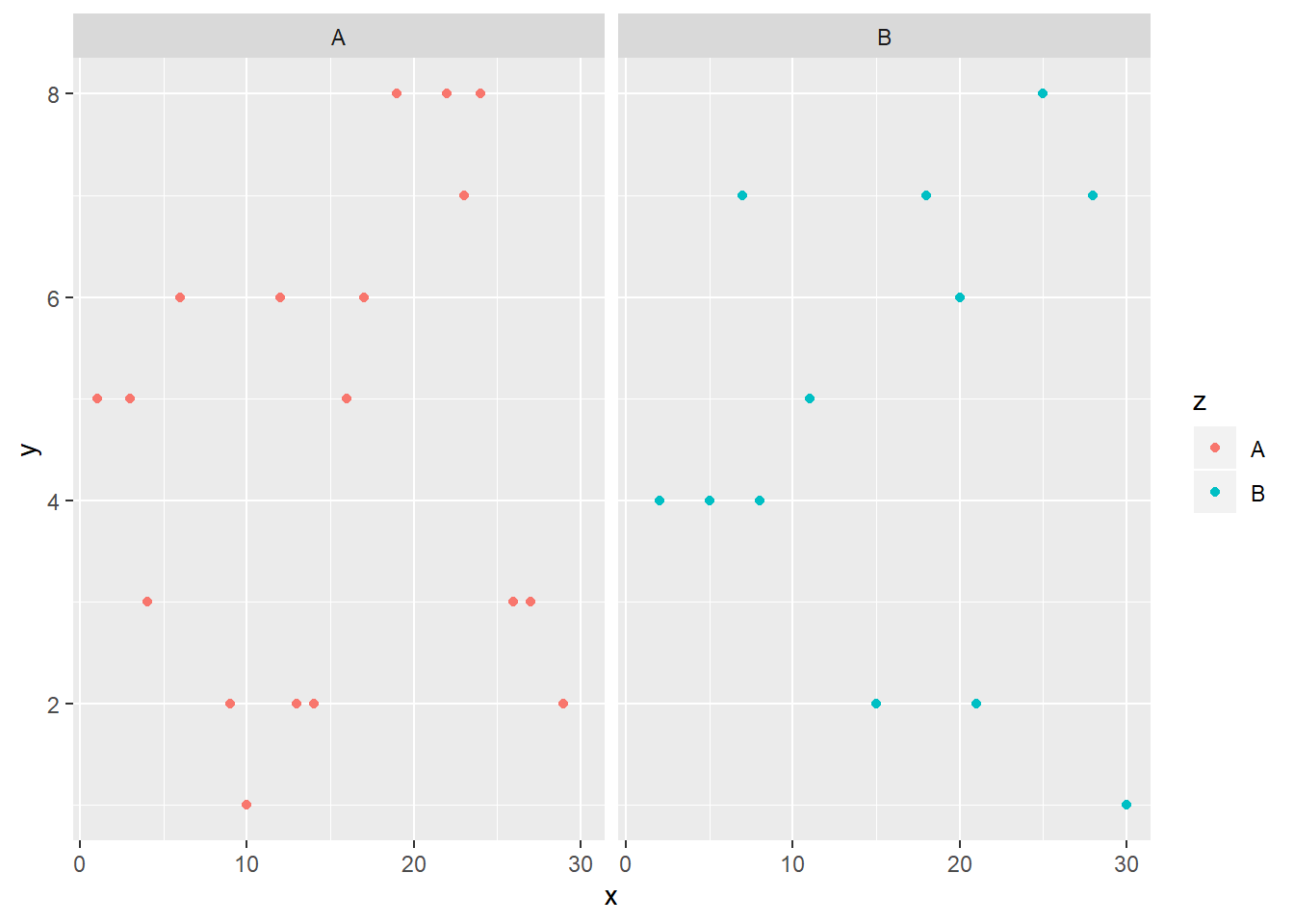The following code makes the two plots top to bottom:

ggplot(data=df)+
aes(x,y, color=z)+
geom_point()+
facet_wrap(~ z, ncol = 1)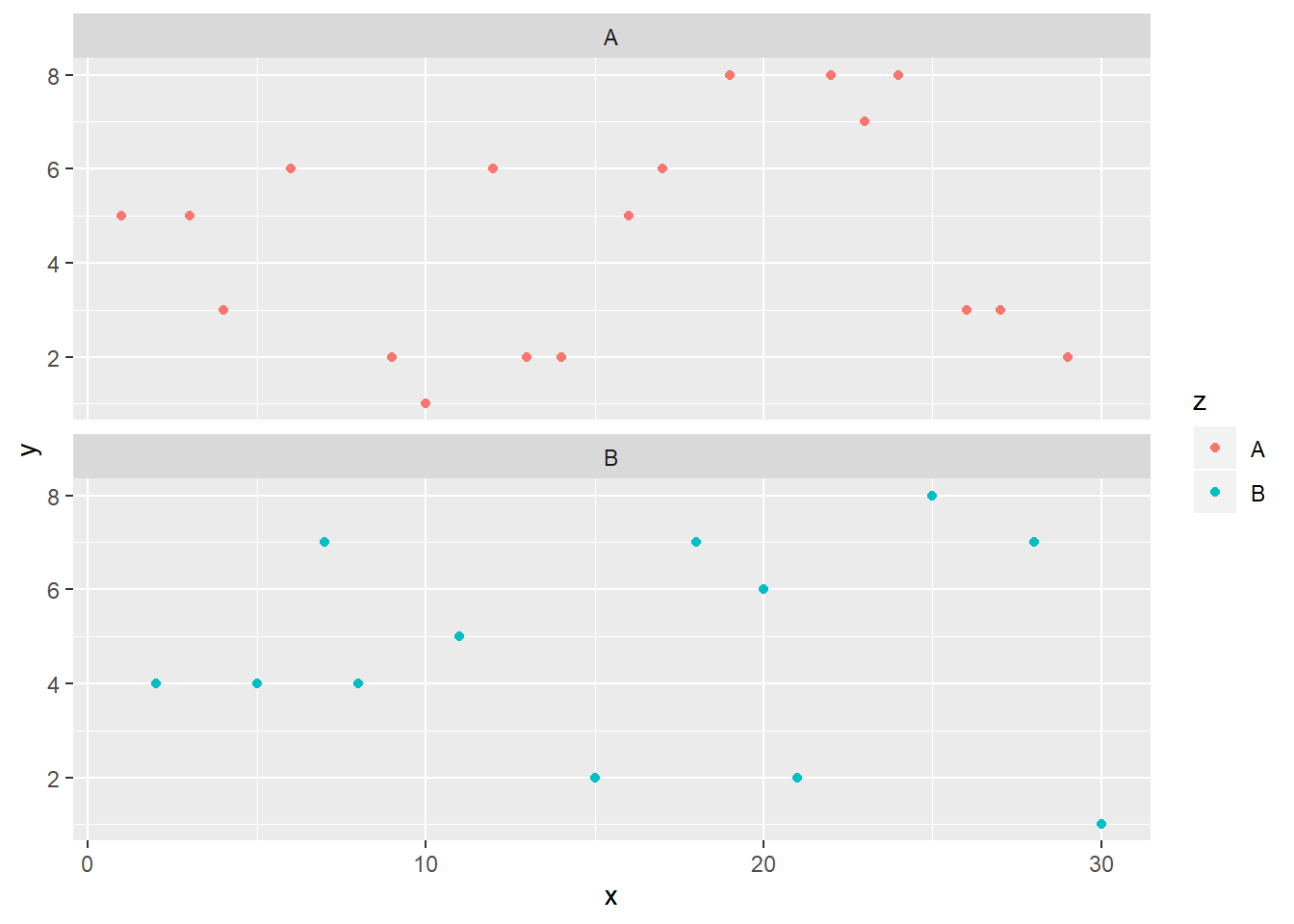### 11.1.6 Basic themes

We can also change the theme of the plot. There are four basic themes.

+theme_grey()
+theme_bw()
+theme_minimal()
+theme_classic()

Basic themes: Grey

ggplot(df)+aes(x,y, color=z)+geom_point()+
theme_grey()Basic themes: BW

ggplot(df)+aes(x,y, color=z)+geom_point()+
theme_bw()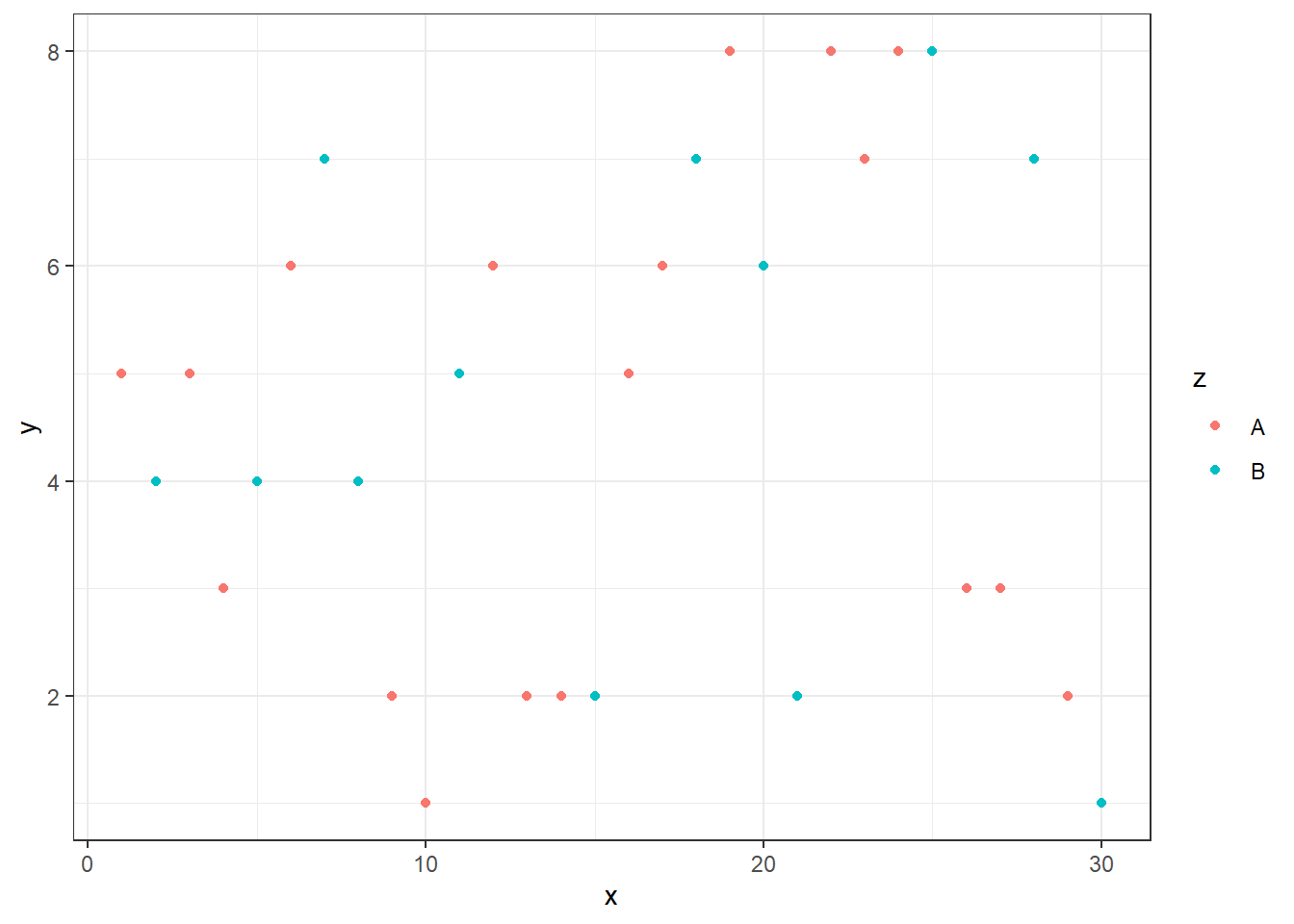Basic themes: minimal

ggplot(df)+aes(x,y, color=z)+geom_point()+
theme_minimal()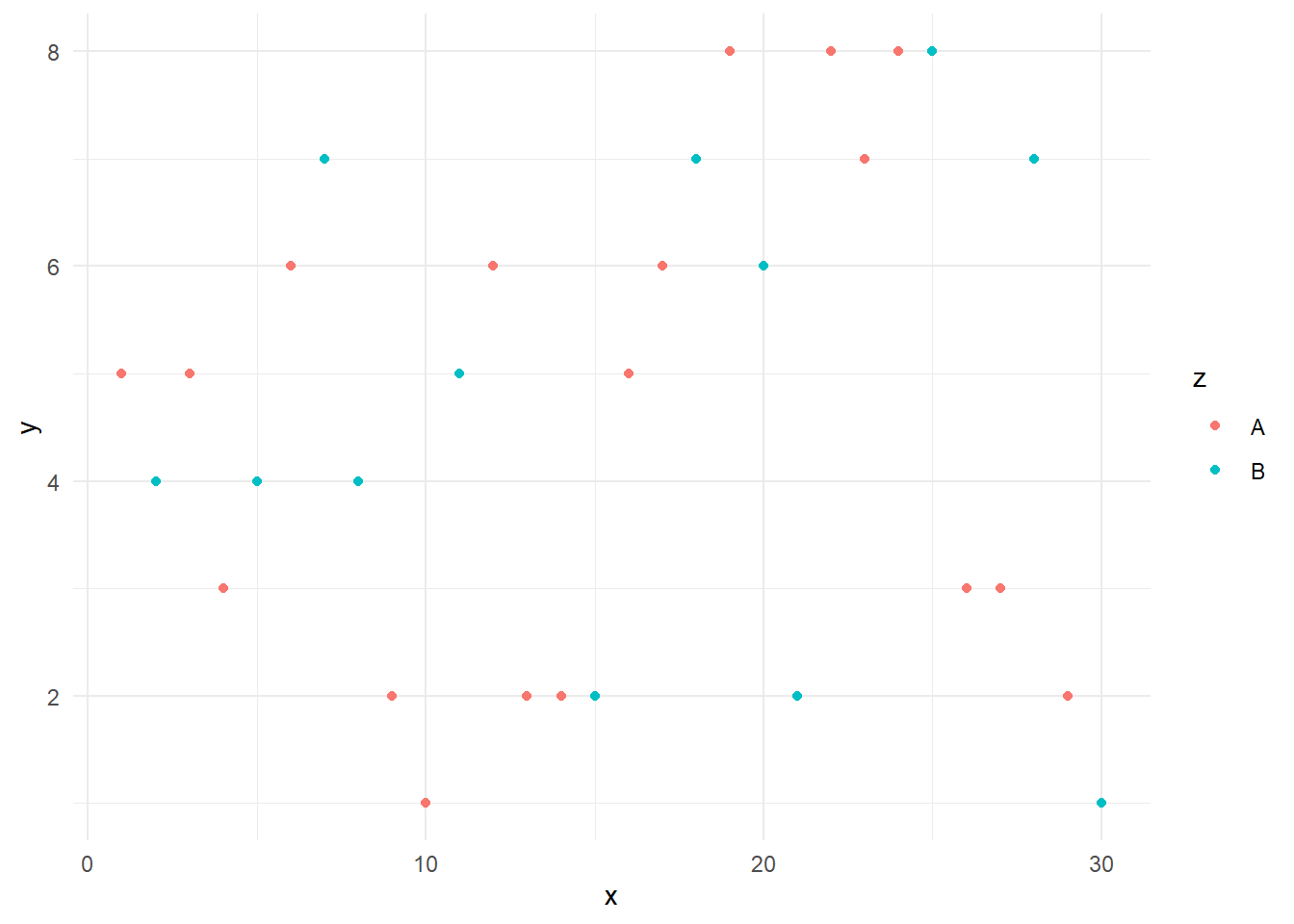Basic themes: classic

ggplot(df)+aes(x,y, color=z)+geom_point()+
theme_classic()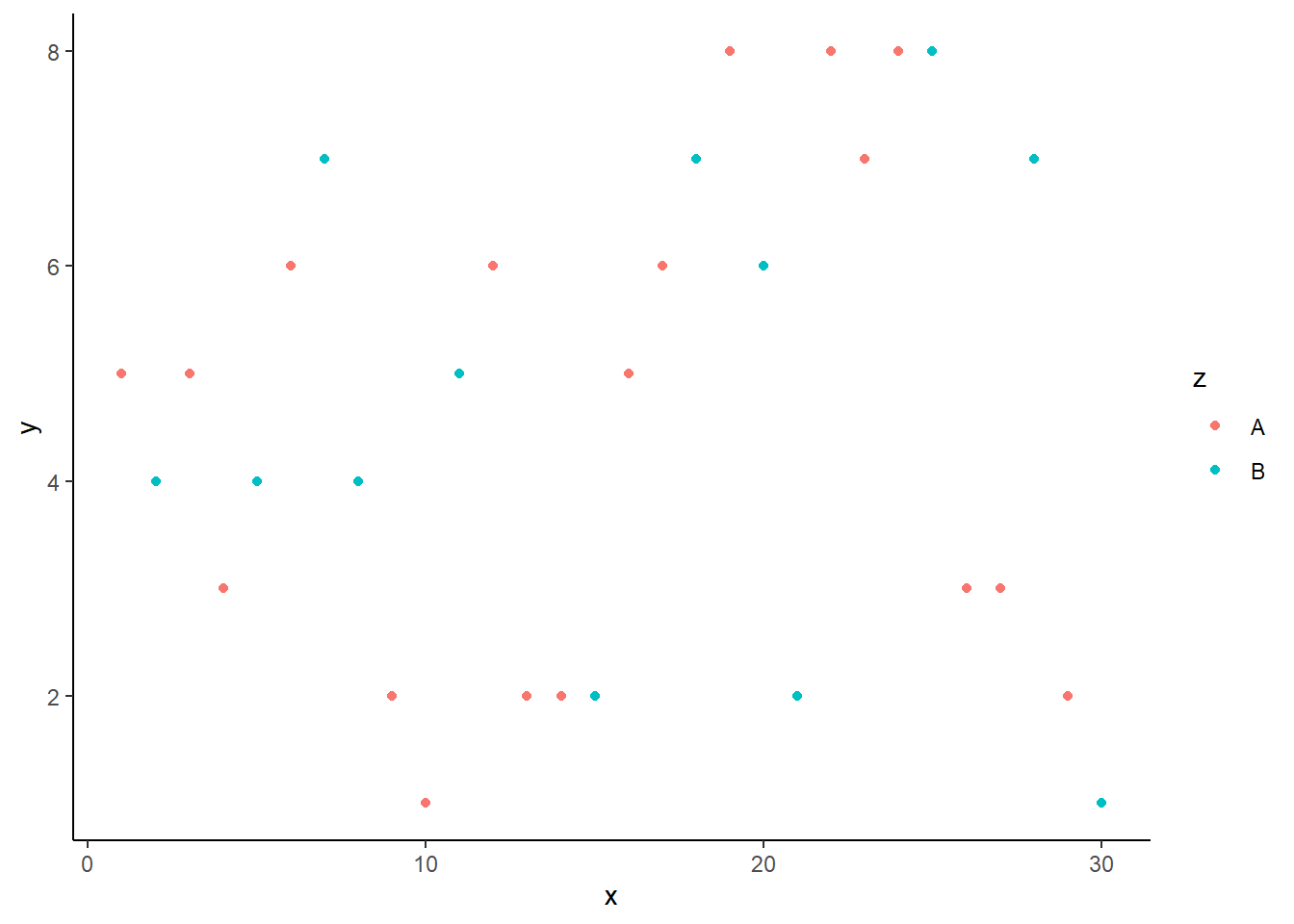### 11.1.7 ggthemes package

Finally, we want to introduce a better theme for plotting. Here, we need to install and load the ggthemes package.

install.packages("ggthemes")
library(ggthemes)

There are three interesting themes:

1. Stata,
2. Excel, and
3. Economist.

To get the Stata theme, we use theme_stata() and scale_colour_stata()

ggplot(df) + aes(x,y, color=z)+ geom_point()+
theme_stata() + scale_colour_stata()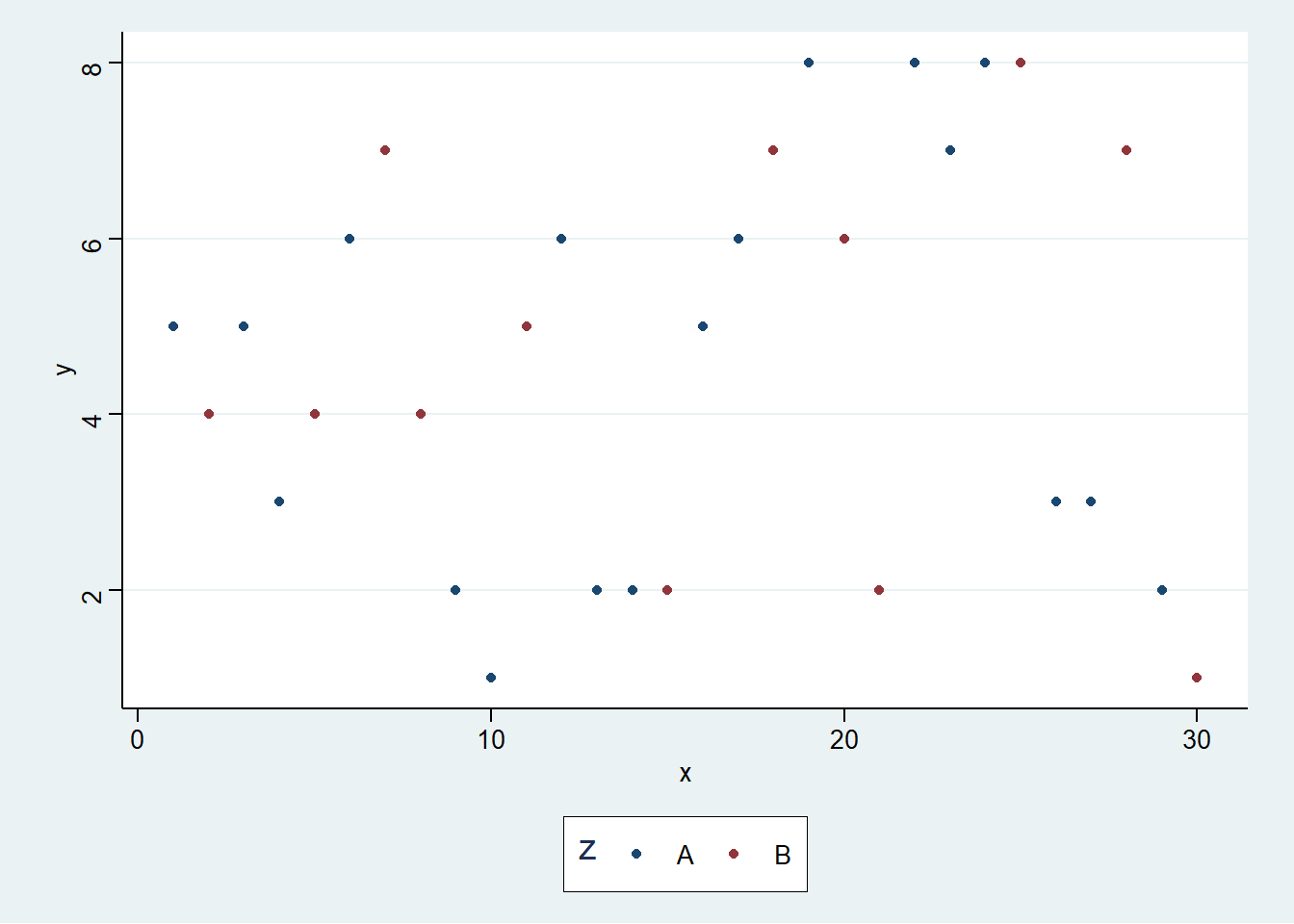To use the Excel theme, we use theme_excel() + scale_colour_excel(). It is not recommended to use it in practice.

ggplot(df) + aes(x,y, color=z)+ geom_point()+
theme_excel() + scale_colour_excel()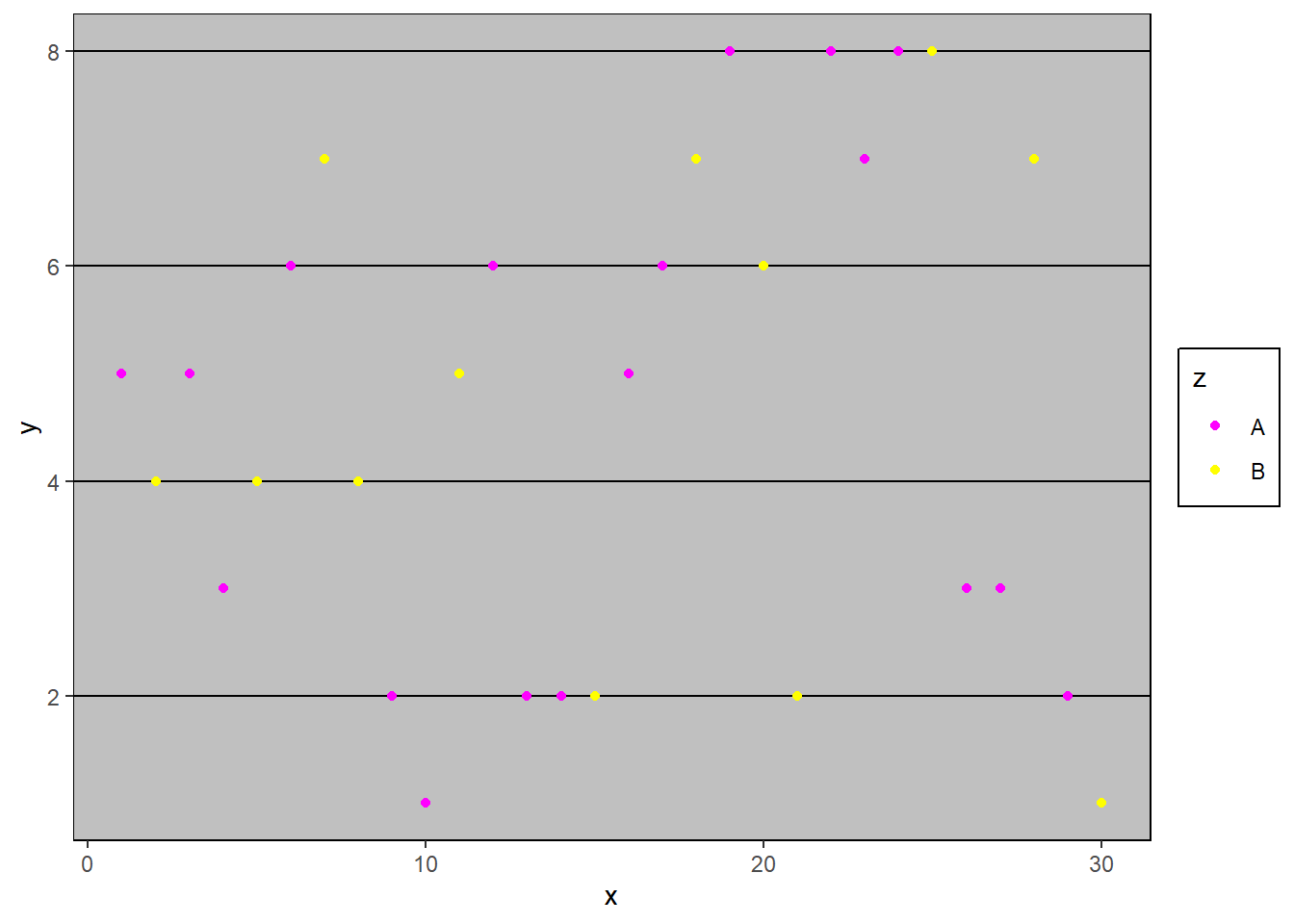To create the theme like the Economist magazine, we can use theme_economist() and scale_colour_economist().

ggplot(df) + aes(x,y, color=z)+ geom_point() +
theme_economist() + scale_colour_economist()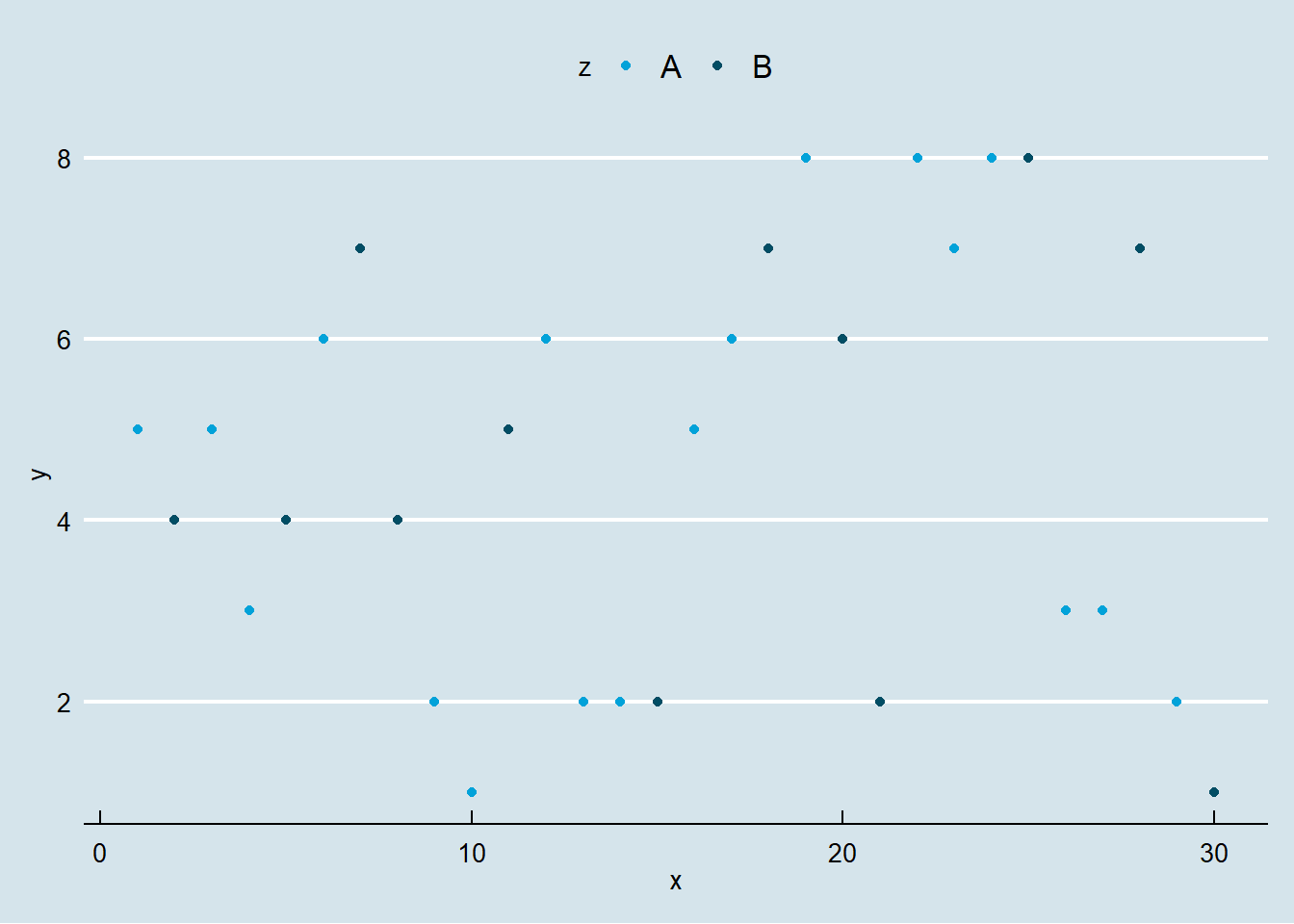Here is the Economist theme with some further decorations.

ggplot(df) +
geom_line(aes(x,y, colour = z), size=1.5)+
theme_economist() + scale_colour_economist()+
theme(legend.position="bottom",
axis.title = element_text(size = 12),
legend.text = element_text(size = 9),
legend.title=element_text(face = "bold",
size = 9)) +
ggtitle("Title")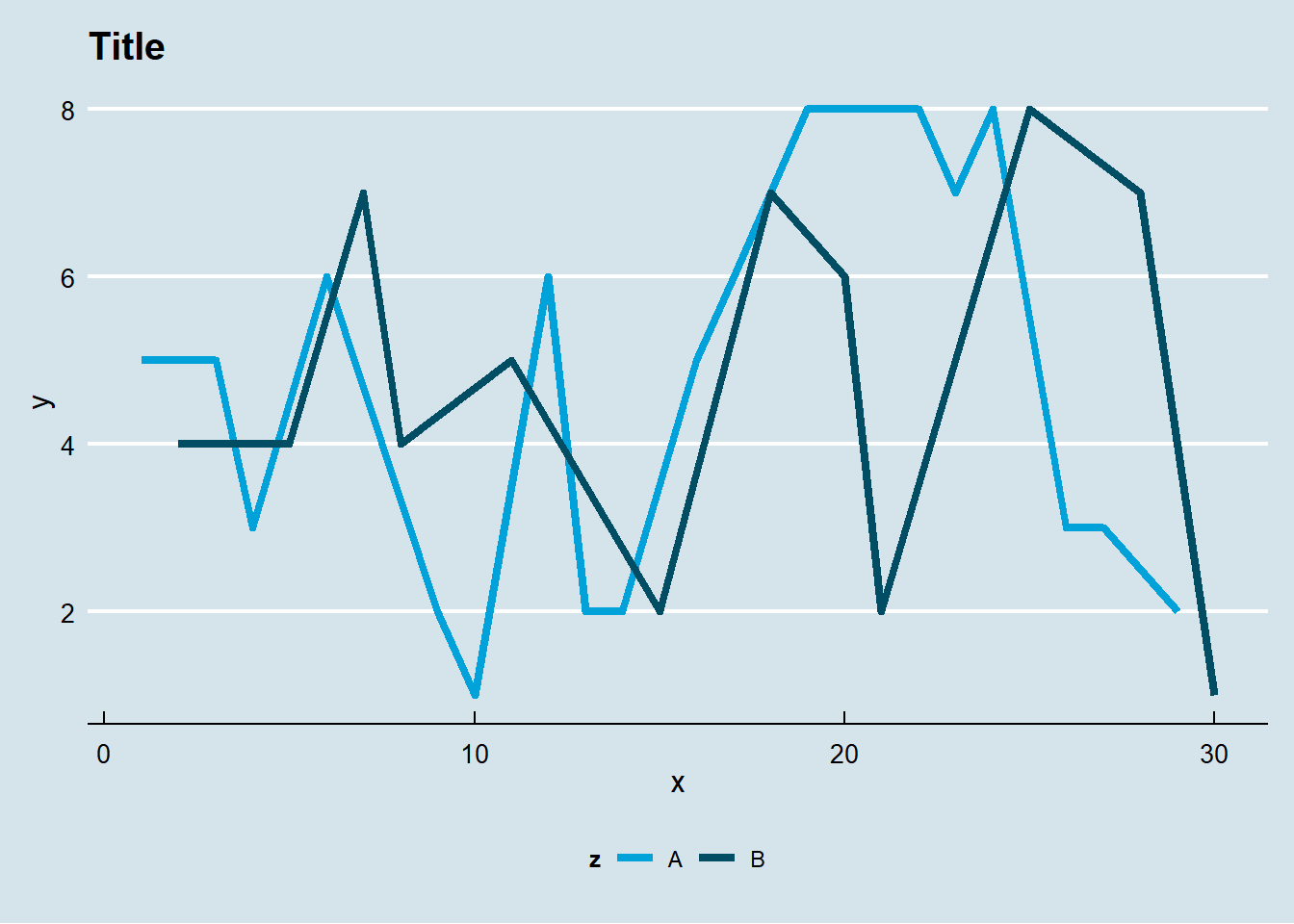### 11.1.8 Application: Worldbank

We want to illustrate our plotting function using data from the World Bank. We will use the wbstats package to download data, and reshape package to convert the data into long format.

library(wbstats)
library(dplyr)
library(reshape)
df<- wb(country=c("US", "SG"),
indicator = c("SP.POP.TOTL",
"NY.GDP.PCAP.KD"),
startdate = 2000, enddate = 2017)
df <- dplyr::select(df, date, indicator,
country,value)
temp <- melt(df,
id=c("date","indicator","country"))
charts.data <- cast(temp, country + date~indicator)
colnames(charts.data) <- c("country", "year","gdp", "pop")
charts.data$year <- as.numeric(charts.data$year)

Now we are ready to plot the graph.

p1<-ggplot()+
geom_line(aes(x = year,y = gdp,color = country),
size=1.5, data = charts.data)+
theme_economist() + scale_colour_economist() +
theme(legend.position="bottom",
axis.title = element_text(size = 12),
legend.text = element_text(size = 9),
legend.title=element_text(face = "bold", size = 9)) +
ggtitle("GDP per capita (US\$)")
print(p1)